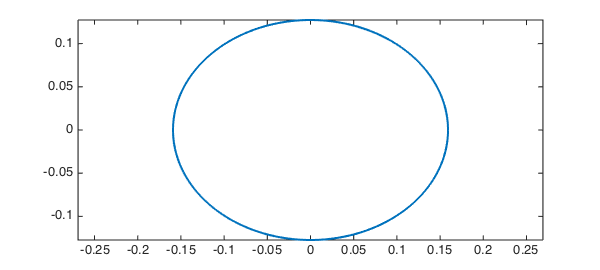The ellipse we use is the one used by Poisson in his paper of 1827 , with semiaxis lengths $0.5/\pi$ and $0.4/\pi$. We know thanks to M. Poisson that the perimeter is $0.902779927$ [*].

exact = 0.90277992777219;

We now attempt to recompute this value using Chebfun.

theta = chebfun(@(theta) theta,[0,2*pi]);
x = (0.5/pi)*cos(theta);
y = (0.4/pi)*sin(theta);
plot(x,y,'-','LineWidth',2), axis equal
arc_length = norm(sqrt(diff(x).^2+diff(y).^2),1)
arc_length =
0.902779927772194[*] Confusingly Poisson reported this number with a misprint in the 2nd place!

       "la valeur approchee de I sera I = 0,9927799272"
1. S.-D. Poisson, Sur le calcul numerique des integrales definies, Memoires de L'Academie Royale des Sciences de L'Institut de France 4 (1827), pp. 571-602 (written in 1823).